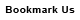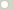# kilogram-force centimeter (Torque, Metric)

## 1 kilogram-force centimeter is equal to:Metric newton meter 0.0980665012480924 newton centimeter 9.80665012480924 dyne meter 9806.65012480924 dyne centimeter 980665.012480924 kilogram-force meter 0.01 gram-force meter 10 gram-force centimeter 1000 British and U.S. long ton-force foot 3.229024e-05 short ton-force foot 3.6165069e-05 pound-force foot 0.072330139 pound-force inch 0.86796166 ounce-force inch 13.887387ConversionsAreaCapacity and VolumeCircular measureComputer storageCurrencyDistance and LengthEnergy and WorkFuel ConsumptionMass and WeightPowerPressureSpeedTemperatureTimeTorqueSearchLink to us